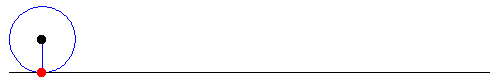# Cycloid

Calculus Level 3The parametric equation of a cycloid is given below.

$\large x = a(t - \sin t) \\ \large y = a(1 - \cos t)$

What is the area of the region bounded by the two arcs of the cycloid in the above figure?

×